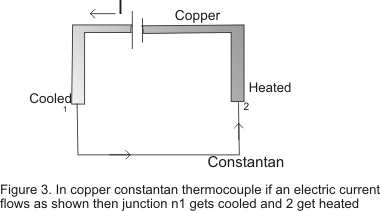# Peltier effect

## Peltier Effect

• Peltier effect is named after his discover Jean Peltier who in 1934 discovered a thermo-electric effect which is converse of Seaback effect
• Peltier discovered that "when an electric current is passed through two dissimilar conductor connected to form a thermo-couple ,heat is evolved at one junction and absorbed at the other end.The absorption and evolution of heat depends on the direction of flow of current• Peltier effect is entirely reversible in nature

Peltier Coefficient

• Peltier coefficient is defined as the amount of heat energy absorbed or evolved due to Peltier effect at the junction of two dissimilar metals when one coulomb of charge passes through the junction
• Peltier coefficient is denoted by π
• Value of Peltier Coefficient is different for different thermo-couple .Its value also depends upon the temperature of the junction
• If q amount of charge passes through the junction then
Energy absorbed or evolved=πq
if V is the contact Potential difference
Then work done=qV
Now heat absorbed=Work done
So π=V
• Hence Peltier coefficient (in J/C) at a junction is numerically equal to the contact v in(Volts)